TitleCollege Algebra
Tutorial 18: Solving Polynomial Equations by Factoring

WTAMU > Virtual Math Lab > College AlgebraLearning Objectives

 After completing this tutorial, you should be able to: Write a polynomial equation in standard form. Use the zero factor property. Solve polynomial equations by factoring.Introduction

 In this tutorial we will be putting our factoring skills to the test again.  We will be looking at solving polynomial equations, which include quadratic equations, by factoring.   After completing this tutorial, you will be a master at solving polynomial equations.  Solving equations in general is a very essential part of algebra.  So I guess we better get to it.Tutorial

 Polynomial Equation

 A polynomial equation is one polynomial set equal to another polynomial. If you need a review on polynomials, feel free to go to Tutorial 6: Polynomials. The following is an example of a polynomial equation: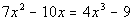Standard Form of a Polynomial Equation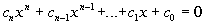When a polynomial is set equal to 0 and is written in descending order by degrees of the terms, the equation is in standard form. The following is an example of a polynomial equation in standard form: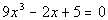A quadratic equation is a special type of polynomial equation, one where the polynomial is of degree 2.  If you need a review on quadratic equations feel free to go to Tutorial 17: Quadratic Equations. Recall that a quadratic equation in standard form looks like this: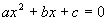.

 Solving Polynomial Equations by Factoring

Note that the steps are essentially the same as when you solve a quadratic equation by factoring, which is shown in Tutorial 17: Quadratic Equations.

Step 1: Simplify each side if needed.

 This would involve things like removing ( ), removing fractions, adding like terms, etc.  To remove ( ):  Just use the distributive property. To remove fractions: Since fractions are another way to write division, and the inverse of divide is to multiply, you remove fractions by multiplying both sides by the LCD of all of your fractions.

Step 2: Write in standard form,, if needed.

 If it is not in standard form, move any term(s) to the appropriate side by using the addition/subtraction property of equality.  Also, make sure that it is written in descending order by degrees of the terms.

Step 3: Factor.

 If you need a review on factoring go to Tutorial 7: Factoring Polynomials.

Step 4:  Use the Zero-Product Principle.

 If ab = 0, then a = 0 or b = 0.  0 is our magic number because the only way a product can become 0 is if at least one of it's factors is 0.  You can not guarantee what the factors would have to be if the product was set equal to any other number.  For example if ab = 1, then a = 5 and b = 1/5 or a = 3 and b = 1/3, etc.  But with the product set equal to 0, we can guarantee finding the solution by setting each factor equal to 0.  That is why it is important to get it in standard form to begin with.

Step 5: Solve for the equation(s) set up in step 4.Example 1: Solve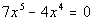by factoring.

 Step 1: Simplify each side if needed.

 This polynomial equation is already simplified.

 Step 2: Write in standard form,, if needed.

 This polynomial equation is already in standard form.

 Step 3: Factor.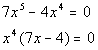*Poly. eq. in standard form *Factor out the GCF

 Step 4:  Use the Zero-Product Principle AND Step 5: Solve for the equation(s) set up in step 4.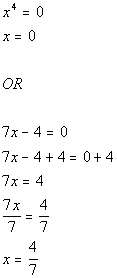*Use Zero-Product Principle *Set 1st factor = 0 and solve       *Set 2nd factor = 0 and solve

 There are two solutions to this polynomial equation: x = 0 and x = 4/7.Example 2: Solve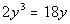by factoring.

 Step 1: Simplify each side if needed.

 This polynomial equation is already simplified.

 Step 2: Write in standard form,, if needed.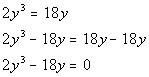*Inverse of add. 18y is sub. 18y *Poly. eq. in standard form

 Step 3: Factor.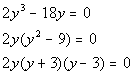*Poly. eq. in standard form *Factor out the GCF *Factor a  diff. of squares

 Step 4:  Use the Zero-Product Principle AND Step 5: Solve for the equation(s) set up in step 4.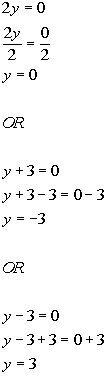*Use Zero-Product Principle *Set 1st factor = 0 and solve           *Set 2nd factor = 0 and solve             *Set 3rd factor = 0 and solve

 There are three solutions to this polynomial equation: y = 0, y = -3 and y = 3.Example 3: Solve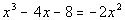by factoring.

 Step 1: Simplify each side if needed.

 This polynomial equation is already simplified.

 Step 2: Write in standard form,, if needed.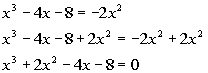*Inverse of sub. 2x^2 is add. 2x^2 *Poly. eq. in standard form

 Step 3: Factor.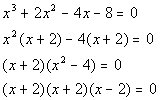*Poly. eq. in standard form *Factor by grouping

 Step 4:  Use the Zero-Product Principle AND Step 5: Solve for the equation(s) set up in step 4.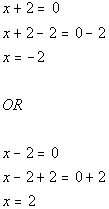*Use Zero-Product Principle *Set 1st factor = 0 and solve *Note that the 2nd factor is the same as the 1st one         *Set 3rd factor = 0 and solve

 There are two solutions to this polynomial equation: x = -2 and x = 2.Practice Problems

 These are practice problems to help bring you to the next level.  It will allow you to check and see if you have an understanding of these types of problems. Math works just like anything else, if you want to get good at it, then you need to practice it.  Even the best athletes and musicians had help along the way and lots of practice, practice, practice, to get good at their sport or instrument.  In fact there is no such thing as too much practice. To get the most out of these, you should work the problem out on your own and then check your answer by clicking on the link for the answer/discussion for that  problem.  At the link you will find the answer as well as any steps that went into finding that answer.Practice Problems 1a - 1b: Solve each polynomial equation by factoring.

 1a.(answer/discussion to 1a) 1b.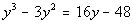(answer/discussion to 1b)Need Extra Help on these Topics?

The following is a webpage that can assist you in the topics that were covered on this page:

 http://www.wtamu.edu/academic/anns/mps/math/mathlab/int_algebra/int_alg_tut30_eqfact.htm This webpage helps you with solving polynomial equations by factoring.

Go to Get Help Outside the Classroom found in Tutorial 1: How to Succeed in a Math Class for some more suggestions.

WTAMU > Virtual Math Lab > College Algebra

Videos at this site were created and produced by Kim Seward and Virginia Williams Trice.
Last revised on Dec. 16, 2009 by Kim Seward.